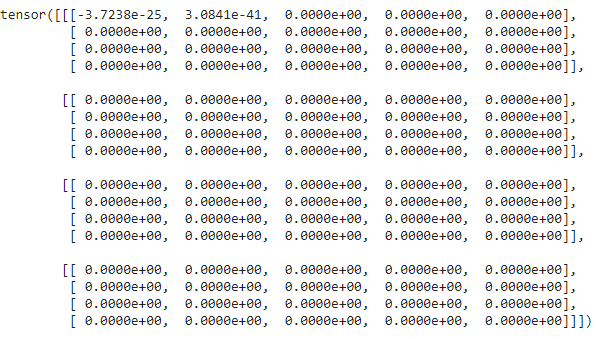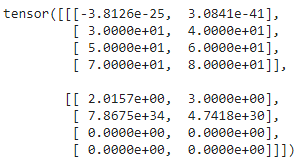Open in App
Not now

# Reshaping a Tensor in Pytorch

• Last Updated : 01 Sep, 2021

In this article, we will discuss how to reshape a Tensor in Pytorch. Reshaping allows us to change the shape with the same data and number of elements as self but with the specified shape, which means it returns the same data as the specified array, but with different specified dimension sizes.

Creating Tensor for demonstration:

Python code to create a 1D Tensor and display it.

## Python3

 `# import torch module``import` `torch` `# create an 1 D etnsor with 8 elements``a ``=` `torch.tensor([``1``,``2``,``3``,``4``,``5``,``6``,``7``,``8``])` `# display tensor shape``print``(a.shape)` `# display tensor``a`

Output:

```torch.Size()
tensor([1, 2, 3, 4, 5, 6, 7, 8])```

## Method 1 : Using reshape() Method

This method is used to reshape the given tensor into a given shape( Change the dimensions)

Syntax: tensor.reshape([row,column])

where,

• tensor is the input tensor
• row represents the number of rows in the reshaped tensor
• column represents the number of columns  in the reshaped tensor

Example 1: Python program to reshape a 1 D tensor to a two-dimensional tensor.

## Python3

 `# import torch module``import` `torch` `# create an 1 D etnsor with 8 elements``a ``=` `torch.tensor([``1``, ``2``, ``3``, ``4``, ``5``, ``6``, ``7``, ``8``])` `# display tensor shape``print``(a.shape)` `# display  actual tensor``print``(a)` `# reshape tensor into 4 rows and 2 columns``print``(a.reshape([``4``, ``2``]))` `# display shape of reshaped tensor``print``(a.shape)`

Output:

```torch.Size()
tensor([1, 2, 3, 4, 5, 6, 7, 8])
tensor([[1, 2],
[3, 4],
[5, 6],
[7, 8]])
torch.Size()```

Example 2: Python code to reshape tensors into 4 rows and 2 columns

## Python3

 `# import torch module``import` `torch` `# create an 1 D etnsor with 8 elements``a ``=` `torch.tensor([``1``, ``2``, ``3``, ``4``, ``5``, ``6``, ``7``, ``8``])` `# display tensor shape``print``(a.shape)` `# display  actual tensor``print``(a)` `# reshape tensor into 4 rows and 2 columns``print``(a.reshape([``4``, ``2``]))` `# display shape``print``(a.shape)`

Output:

```torch.Size()
tensor([1, 2, 3, 4, 5, 6, 7, 8])
tensor([[1, 2],
[3, 4],
[5, 6],
[7, 8]])
torch.Size()```

Example 3: Python code to reshape tensor into 8 rows and 1 column.

## Python3

 `# import torch module``import` `torch` `# create an 1 D etnsor with 8 elements``a ``=` `torch.tensor([``1``, ``2``, ``3``, ``4``, ``5``, ``6``, ``7``, ``8``])` `# display tensor shape``print``(a.shape)` `# display  actual tensor``print``(a)` `# reshape tensor into 8 rows and 1 column``print``(a.reshape([``8``, ``1``]))` `# display shape``print``(a.shape)`

Output:

```torch.Size()
tensor([1, 2, 3, 4, 5, 6, 7, 8])
tensor([,
,
,
,
,
,
,
])
torch.Size()```

## Method 2 : Using flatten() method

flatten() is used to flatten an N-Dimensional tensor to a 1D Tensor.

Syntax: torch.flatten(tensor)

Where, tensor is the input tensor

Example 1: Python code to create a tensor  with 2 D elements and flatten this vector

## Python3

 `# import torch module``import` `torch` `# create an 2 D tensor with 8 elements each``a ``=` `torch.tensor([[``1``,``2``,``3``,``4``,``5``,``6``,``7``,``8``],``                  ``[``1``,``2``,``3``,``4``,``5``,``6``,``7``,``8``]])` `# display actual tensor``print``(a)` `# flatten a tensor with flatten() function``print``(torch.flatten(a))`

Output:

```tensor([[1, 2, 3, 4, 5, 6, 7, 8],
[1, 2, 3, 4, 5, 6, 7, 8]])
tensor([1, 2, 3, 4, 5, 6, 7, 8, 1, 2, 3, 4, 5, 6, 7, 8])```

Example 2: Python code to create a tensor  with 3 D elements and flatten this vector

## Python3

 `# import torch module``import` `torch` `# create an 3 D tensor with 8 elements each``a ``=` `torch.tensor([[[``1``,``2``,``3``,``4``,``5``,``6``,``7``,``8``],``                 ``[``1``,``2``,``3``,``4``,``5``,``6``,``7``,``8``]],``                ``[[``1``,``2``,``3``,``4``,``5``,``6``,``7``,``8``],``                 ``[``1``,``2``,``3``,``4``,``5``,``6``,``7``,``8``]]])` `# display actual tensor``print``(a)` `# flatten a tensor with flatten() function``print``(torch.flatten(a))`

Output:

tensor([[[1, 2, 3, 4, 5, 6, 7, 8],

[1, 2, 3, 4, 5, 6, 7, 8]],

[[1, 2, 3, 4, 5, 6, 7, 8],

[1, 2, 3, 4, 5, 6, 7, 8]]])

tensor([1, 2, 3, 4, 5, 6, 7, 8, 1, 2, 3, 4, 5, 6, 7, 8, 1, 2, 3, 4, 5, 6, 7, 8,

1, 2, 3, 4, 5, 6, 7, 8])

## Method 3: Using view() method

view() is used to change the tensor in two-dimensional format IE rows and columns. We have to specify the number of rows and the number of columns to be viewed.

Syntax: tensor.view(no_of_rows,no_of_columns)

where,

• tensor is an input one dimensional tensor
• no_of_rows is the total number of the rows that the tensor is viewed
• no_of_columns is the total number of the columns  that the tensor is viewed.

Example 1: Python program to create a tensor with 12 elements and view with 3 rows and 4 columns and vice versa.

## Python3

 `# importing torch module``import` `torch` `# create one dimensional tensor 12 elements``a``=``torch.FloatTensor([``24``, ``56``, ``10``, ``20``, ``30``,``                     ``40``, ``50``, ``1``, ``2``, ``3``, ``4``, ``5``]) ` `# view tensor in 4 rows and 3 columns``print``(a.view(``4``, ``3``))`` ` `# view tensor in 3 rows and 4 columns``print``(a.view(``3``, ``4``))`

Output:

```tensor([[24., 56., 10.],
[20., 30., 40.],
[50.,  1.,  2.],
[ 3.,  4.,  5.]])
tensor([[24., 56., 10., 20.],
[30., 40., 50.,  1.],
[ 2.,  3.,  4.,  5.]])```

Example 2: Python code to change the view of a tensor into 10 rows and one column and vice versa.

## Python3

 `# importing torch module``import` `torch` `# create one dimensional tensor 10 elements``a ``=` `torch.FloatTensor([``24``, ``56``, ``10``, ``20``, ``30``,``                     ``40``, ``50``, ``1``, ``2``, ``3``]) ` `# view tensor in 10 rows and 1 column``print``(a.view(``10``, ``1``))`` ` `# view tensor in 1 row and 10 columns``print``(a.view(``1``, ``10``))`

Output:

```tensor([[24.],
[56.],
[10.],
[20.],
[30.],
[40.],
[50.],
[ 1.],
[ 2.],
[ 3.]])
tensor([[24., 56., 10., 20., 30., 40., 50.,  1.,  2.,  3.]])```

## Method 4: Using resize() method

This is used to resize the dimensions of the given tensor.

Syntax: tensor.resize_(no_of_tensors,no_of_rows,no_of_columns)

where:

• tensor is the input tensor
• no_of_tensors represents the total number of tensors to be generated
• no_of_rows represents the total number of rows in the new resized tensor
• no_of_columns represents the total number of columns in the new resized tensor

Example 1: Python code to create an empty one D tensor and create 4 new tensors with 4 rows and 5 columns

## Python3

 `# importing torch module``import` `torch` `# create one dimensional tensor``a ``=` `torch.Tensor() ` `# resize the tensor to 4 tensors.``# each tensor with 4 rows and 5 columns``print``(a.resize_(``4``, ``4``, ``5``))`

Output:Example 2: Create a 1 D tensor with elements and resize to 3 tensors with 2 rows and 2 columns

## Python3

 `# importing torch module``import` `torch` `# create one dimensional``a ``=` `torch.Tensor() ` `# resize the tensor to 2 tensors.``# each tensor with 4 rows and 2 columns``print``(a.resize_(``2``, ``4``, ``2``))`

Output:## Method 5: Using unsqueeze() method

This is used to reshape a tensor by adding new dimensions at given positions.

Syntax: tensor.unsqueeze(position)

where, position is the dimension index which will start from 0.

Example 1: Python code to create 2 D tensors and add a dimension in 0 the dimension.

## Python3

 `# importing torch module``import` `torch` `# create two dimensional tensor``a ``=` `torch.Tensor([[``2``,``3``], [``1``,``2``]]) ` `# display shape``print``(a.shape)` `# add dimension at 0 position``added ``=` `a.unsqueeze(``0``)` `print``(added.shape)`

Output:

```torch.Size([2, 2])
torch.Size([1, 2, 2])```

Example 2: Python code to create 1 D tensor and add dimensions

## Python3

 `# importing torch module``import` `torch` `# create one dimensional tensor``a ``=` `torch.Tensor([``1``, ``2``, ``3``, ``4``, ``5``]) ` `# display shape``print``(a.shape)` `# add dimension at 0 position``added ``=` `a.unsqueeze(``0``)` `print``(added.shape)` `# add dimension at 1 position``added ``=` `a.unsqueeze(``1``)` `print``(added.shape)`

Output:

```torch.Size()
torch.Size([1, 5])
torch.Size([5, 1])```

My Personal Notes arrow_drop_up